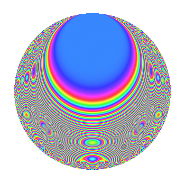# Properties

 Label 804.2.q.bLevel 804 Weight 2 Character orbit 804.q Analytic conductor 6.420 Analytic rank 0 Dimension 60 CM No

# Related objects

## Newspace parameters

 Level: $$N$$ = $$804 = 2^{2} \cdot 3 \cdot 67$$ Weight: $$k$$ = $$2$$ Character orbit: $$[\chi]$$ = 804.q (of order $$11$$ and degree $$10$$)

## Newform invariants

 Self dual: No Analytic conductor: $$6.41997232251$$ Analytic rank: $$0$$ Dimension: $$60$$ Relative dimension: $$6$$ over $$\Q(\zeta_{11})$$ Sato-Tate group: $\mathrm{SU}(2)[C_{11}]$

## $q$-expansion

The dimension is sufficiently large that we do not compute an algebraic $$q$$-expansion, but we have computed the trace expansion.

 $$\operatorname{Tr}(f)(q) =$$ $$60q + 6q^{3} + 2q^{5} + 2q^{7} - 6q^{9} + O(q^{10})$$ $$\operatorname{Tr}(f)(q) =$$ $$60q + 6q^{3} + 2q^{5} + 2q^{7} - 6q^{9} - 11q^{11} - 2q^{13} + 9q^{15} + 21q^{17} + 10q^{19} - 2q^{21} - 10q^{23} - 36q^{25} + 6q^{27} + 4q^{29} - 24q^{31} - 32q^{35} + 2q^{37} + 2q^{39} + 10q^{41} + 23q^{43} + 2q^{45} + 66q^{47} + 34q^{49} + 23q^{51} - 13q^{53} + 27q^{55} + q^{57} + 35q^{59} + 56q^{61} - 9q^{63} + 48q^{65} + 13q^{67} + 10q^{69} + 76q^{71} - q^{73} + 36q^{75} - 38q^{77} - 46q^{79} - 6q^{81} - 26q^{83} + 42q^{85} + 7q^{87} + 58q^{89} - 40q^{91} - 9q^{93} - 29q^{95} - 46q^{97} + O(q^{100})$$

## Embeddings

For each embedding $$\iota_m$$ of the coefficient field, the values $$\iota_m(a_n)$$ are shown below.

For more information on an embedded modular form you can click on its label.

Label $$a_{2}$$ $$a_{3}$$ $$a_{4}$$ $$a_{5}$$ $$a_{6}$$ $$a_{7}$$ $$a_{8}$$ $$a_{9}$$ $$a_{10}$$
25.1 0 0.654861 0.755750i 0 −3.19644 + 2.05423i 0 −0.0504230 0.350699i 0 −0.142315 0.989821i 0
25.2 0 0.654861 0.755750i 0 −1.48760 + 0.956023i 0 −0.105071 0.730785i 0 −0.142315 0.989821i 0
25.3 0 0.654861 0.755750i 0 −0.494572 + 0.317842i 0 0.597152 + 4.15328i 0 −0.142315 0.989821i 0
25.4 0 0.654861 0.755750i 0 1.12656 0.723994i 0 −0.202268 1.40680i 0 −0.142315 0.989821i 0
25.5 0 0.654861 0.755750i 0 1.69814 1.09133i 0 −0.693468 4.82317i 0 −0.142315 0.989821i 0
25.6 0 0.654861 0.755750i 0 2.59336 1.66665i 0 0.180977 + 1.25872i 0 −0.142315 0.989821i 0
193.1 0 0.654861 + 0.755750i 0 −3.19644 2.05423i 0 −0.0504230 + 0.350699i 0 −0.142315 + 0.989821i 0
193.2 0 0.654861 + 0.755750i 0 −1.48760 0.956023i 0 −0.105071 + 0.730785i 0 −0.142315 + 0.989821i 0
193.3 0 0.654861 + 0.755750i 0 −0.494572 0.317842i 0 0.597152 4.15328i 0 −0.142315 + 0.989821i 0
193.4 0 0.654861 + 0.755750i 0 1.12656 + 0.723994i 0 −0.202268 + 1.40680i 0 −0.142315 + 0.989821i 0
193.5 0 0.654861 + 0.755750i 0 1.69814 + 1.09133i 0 −0.693468 + 4.82317i 0 −0.142315 + 0.989821i 0
193.6 0 0.654861 + 0.755750i 0 2.59336 + 1.66665i 0 0.180977 1.25872i 0 −0.142315 + 0.989821i 0
241.1 0 −0.415415 + 0.909632i 0 −3.70609 1.08821i 0 1.46290 + 1.68828i 0 −0.654861 0.755750i 0
241.2 0 −0.415415 + 0.909632i 0 −1.77661 0.521660i 0 0.707286 + 0.816251i 0 −0.654861 0.755750i 0
241.3 0 −0.415415 + 0.909632i 0 −0.960253 0.281956i 0 0.00738542 + 0.00852322i 0 −0.654861 0.755750i 0
241.4 0 −0.415415 + 0.909632i 0 1.61021 + 0.472801i 0 −1.73357 2.00064i 0 −0.654861 0.755750i 0
241.5 0 −0.415415 + 0.909632i 0 1.77448 + 0.521035i 0 −3.02047 3.48581i 0 −0.654861 0.755750i 0
241.6 0 −0.415415 + 0.909632i 0 1.80159 + 0.528994i 0 2.39007 + 2.75829i 0 −0.654861 0.755750i 0
265.1 0 0.142315 0.989821i 0 −1.42271 3.11530i 0 2.11496 + 0.621007i 0 −0.959493 0.281733i 0
265.2 0 0.142315 0.989821i 0 −0.536269 1.17426i 0 −0.878330 0.257901i 0 −0.959493 0.281733i 0
See all 60 embeddings
 $$n$$: e.g. 2-40 or 990-1000 Embeddings: e.g. 1-3 or 685.6 Significant digits: Format: Complex embeddings Normalized embeddings Satake parameters Satake angles

## Inner twists

This newform does not have CM; other inner twists have not been computed.

## Hecke kernels

This newform can be constructed as the kernel of the linear operator $$T_{5}^{60} - \cdots$$ acting on $$S_{2}^{\mathrm{new}}(804, [\chi])$$.